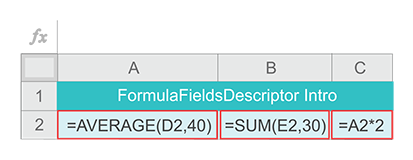Operators Functions API Pricelist Company Blog Contact Us

# FormulaFieldsDescriptor Class

## Introduction

Suppose we have the following three Excel formulas:The below code creates five ComputeFields: inputField1, inputField2, formulaField1, formulaField2 and formulaField3 analogous to input cells D2 and E2, and formula cell A2, B2, and C2. It stores them in the CalculationContext object with corresponding key names. Finally, it invokes the static method compileAllFormulas() to compile formulas in our example and create a FormulaFieldDescription summary of the compilation results:

```ComputeField inputField1 = new InputField(ComputeFieldType.NUMERIC);
ComputeField inputField2 = new InputField(ComputeFieldType.NUMERIC);
ComputeField formulaField1 = new FormulaField(ComputeFieldType.NUMERIC, "AVERAGE(D2,40)");
ComputeField formulaField2 = new FormulaField(ComputeFieldType.NUMERIC, "SUM(E2,30)");
ComputeField formulaField3 = new FormulaField(ComputeFieldType.NUMERIC, "A2*2");
CalculationContext calculationContext = new CalculationContext("FormulaFieldDescriptorIntro");
FormulaFieldsDescriptor formulaFieldsDescriptor;

calculationContext.put("D2", inputField1);
calculationContext.put("E2", inputField2);
calculationContext.put("A2", formulaField1);
calculationContext.put("B2", formulaField2);
calculationContext.put("C2", formulaField3);
formulaFieldsDescriptor = HybridFormulaEvaluator.compileAllFormulas(calculationContext);
```

Excel-like key names for ComputeFields are not required, they are used in this example only for demonstration.

The following JSON object shows the structure and content of the FormulaFieldDescription object created in this example:

• JSON FormulaFieldsDescriptor {7}
• formulaFieldsDependencies 
• A2 
• 0 : C2
• B2 
• (empty array)
• C2 
• (empty array)
• D2 
• 0 : A2
• 1 : C2
• E2 
• 0 : B2
• formulaFieldsEvaluationOrder 
• 0 : B2
• 0 : A2
• 0 : C2
• missingComputeFields 
• (empty array)
• computeFieldsInError 
• (empty array)
• error : false
• missingComputeFieldsIndicator : false
• computeFieldsInErrorIndicator : false

We can observe that:

• A2 and C2 formulas are dependent on the input field D2
• B2 formula is dependent on the input field E2
• C2 formula is dependent on the formula field A2

Such dependencies are used by the ACE4J evaluateDependentFormulas() method.

The order of formulas evaluation is used by the ACE4J evaluateAllFormulas() method. In this example it was determined as {B2, A2, C2}. The order of formulas evaluation is not unique and in this example it can be {B2, A2, C2}, {A2, C2, B2}, or {A2, B2, C2}

## Overview

A FormulaFieldsDescriptor object is created by the compileAllFormulas() method as a summary of its successful or in error results.

The FormulaFieldsDescriptor class provides the following getters:

Compilation related

Map<String, List<String>> getFormulaFieldsDependencies()

List<String> getFormulaFieldsEvaluationOrder()

Compilation and evaluation related

boolean isError()

boolean getMissingComputeFieldsIndicator()

boolean getComputeFieldsInErrorIndicator()

List<String> getMissingComputeFields()

List<String> getComputeFieldsInError()

The evaluateDependentFormulas() and evaluateAllFormulas() methods obtain a FormulaFieldsDescriptor object from the CalculationContext parameter and use it as a data structure, which directs their execution. At the same, time both methods use this object to record all ComputeFields associated with the formulas, that failed to evaluate without errors. They also use this object to record the missing ComputeFields.